#### Introduction

There are many ways that dome-like protrusions can be generated by a displacement shader. This tutorial looks at a couple of procedural techniques that are intended to be a useful basis for a shader that generates water droplets of the type shown shown in figure 1, 2 and 3.Figure 1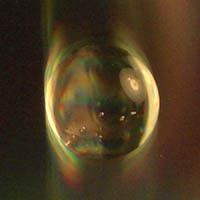Figure 2Figure 3

This tutorial does not consider displacement mapping techniques nor does it address issues of how water droplets might be rendered using a surface shader.

#### Formula

Figure 4 shows the relationship between a line (chord) that cuts a circle. The cord divides the circle into two parts. The lower portion, labelled S for sagitta measures how much the circle "protrudes" beyond the cord. The height of the gray (right-angled triangle) can be calculated from the theorem of Pythagorus ie.

`    sqrt(R * R - L * L)`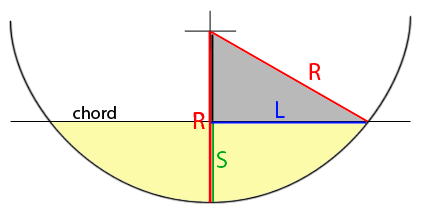Figure 4

Therefore, the sagitta is equal to the radius of the circle less the height of the gray triangle ie.

`    S = R - sqrt(R * R - L * L)`

 This formula forms the basis of another formula called the sphereometer formula that derives its name from the instrument that opticians use when they measure the depth of spherical mirrors.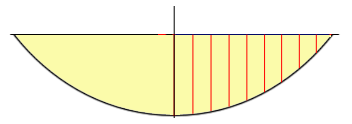Figure 5 The problem with the sphereometer formula is that it only provides a measure of the sagitta - just a single number. A displacement shader, on the other hand, will require a sequence of numbers (figure 5) that measure the depth of the circle at various distances from the central axis of the "dome". Rather than attempting to adapt the formula, a few short-cuts can be taken that that will enable a rounded dome to be produced even though, strickly speaking, it will have a slightly non-circular profile.

#### Squash & Stretch Semi-Circles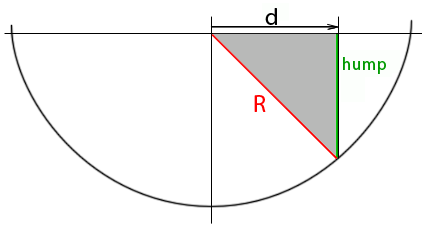Figure 6

If we confine ourselves to a semi-circle of radius "R", then calculating distance "d", figure 6, for a particular value of "r" again can be done using the theorum of Pythagorus (note the subtration) ie.

`    hump = sqrt(R * R - d * d)`

If we do not wish to produce semi-circular dome we can apply a scaling factor (squash/stretch) each time we calculate "d". For our first attempt at applying this to a displacement shader it will be assumed a dome, centered in texture space ie. '`s`' and '`t`' both at 0.5.

Listing 1

 ```/* This shader produces a single bump in the middle of the 'st' texture space. */ displacement dome1(float Km = -2, R = 0.1) { float hump = 0; normal n = normalize(N); float S = (s - 0.5), T = (t - 0.5); float d_sq = S * S + T * T; float R_sq = R * R; /* Ensure the displacement only occurs on the inside of the perimeter of the "R" circle. By using d_sq (d squared) and R_sq (R squared) we need only use the sqrt() function once. */ if(d_sq <= R_sq) hump = sqrt(R_sq - d_sq); P = P - n * hump * Km; N = calculatenormal(P); }```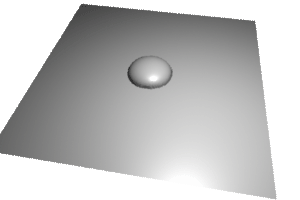Figure 7

Listing 2

 ```/* This shader produces a single bump in the middle of the 'st' texture space. This version of the dome shader allows the user to stretch the dome. */ displacement dome2(float Km = -2, R = 0.2, s_scale = 1, t_scale = 1) { float hump = 0; normal n = normalize(N); /* By applying a scaling factor to either the S or the T the dome can be stretched in either the s or the t direction. */ float S = (s - 0.5) * s_scale, T = (t - 0.5) * t_scale; float d_sq = S * S + T * T; float R_sq = R * R; /* Ensure the displacement only occurs on the inside of the perimeter of the "R" circle. By using d_sq (d squared) and R_sq (R squared) we need only use the sqrt() function once. */ if(d_sq <= R_sq) hump = sqrt(R_sq - d_sq); P = P - n * hump * Km; N = calculatenormal(P); } ```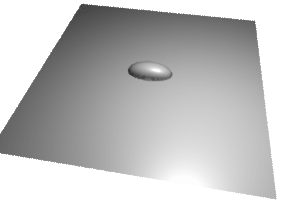Figure 8

Listing 3

 ```Display "untitled" "framebuffer" "rgba" Format 300 200 1 Projection "perspective" "fov" 40 ShadingRate 0.5 Translate 0 0.5 5 Rotate -50 1 0 0 Rotate 10 0 1 0 Scale 1 1 -1 WorldBegin LightSource "pointlight" 1 "intensity" 25 "from" [1 4 1] TransformBegin Surface "plastic" Displacement "dome1" "Km" 2 "R" 0.1 #"s_scale" 1 #"t_scale" 2 Attribute "bound" "displacement" [0.1] Scale 4 4 4 Polygon "P" [-0.5 0 -0.5 0.5 0 -0.5 0.5 0 0.5 -0.5 0 0.5] "st" [0 0 1 0 1 1 0 1] TransformEnd WorldEnd```